The fundamental limitation to high- speed communication systems over the embedded standard single-mode fiber at 1.55 mm is the linear chromatic dispersion.

Typical value of β2  = –20ps2/km at 1.55 µm for SMF leads to D=16 ps/(nm.km). For bit rate B = 40 Gb/s, the slot duration will be TB = 25 ps. If we consider the duty cycle

0.5 → TFWHM  = 12.5ps → T0  = 7.5ps

the corresponding dispersion length will be approximately LD ~ 2.8 km.

This lesson demonstrates the following:

• Compare RZ- and NRZ- modulation format transmission in SMF at 40 Gb/s taking into account: group velocity dispersion, self-phase modulation due to the Kerr nonlinearity, linear losses, and periodical amplification with ASE noise. The large group velocity dispersion is compensated for with a post-dispersion compensation scheme.
• Analyze the influence of accumulated amplifier noise and self-phase modulation for RZ -modulation format transmission in SMF at 40 Gb/s.
• Compare post-dispersion and pre-dispersion compensation schemes for RZ – modulation format transmission in SMF at 40 Gb/s.

Figure 1 and Figure 2 show the layouts used for observing the RZ- and NRZ- modulation formats.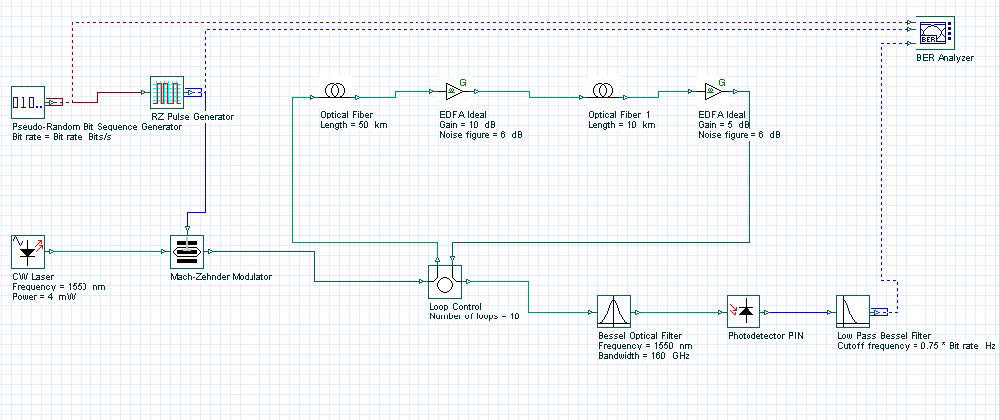Figure 1: RZ layout — modulation formatFigure 2: NRZ layout — modulation format

The setup mode Set bit rate with following global parameters was used:

• Bit rate = 40 Gb/s
• Sequence length = 128 Bits
• Samples per bit = 128

Therefore:

• Number of samples = Sequence length * Samples per bit = 16384
• Time window = Sequence length * Bit slot = 3.2 ns
• Sampling interval = Time window / Number of samples = 0.195 ps
• Sample rate = 1 / the sampling interval = 5.12 THz

There are no changes in pseudo-random bit sequence generators in either layout. The RZ- generator has the following properties:

• Rectangle shape: Gaussian
• Duty cycle = 0.5 bit
• Rise time = 0.15 bit
• Fall time = 0.25 bit

An externally modulated CW Laser with carrier wavelength λ =1550 nm and line width = 0.1 MHz was used as the optical source.

Standard mode optical fiber has the following properties:

Dispersion coefficientDispersion slope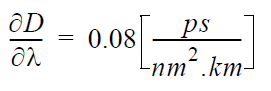Nonlinear coefficient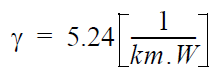Linear losses

α = 0.2 dB/km

Fiber length

LSMF = 50 km

After each segment from SMF, an amplifier compensates the linear losses.

Dispersion compensation fiber has the following properties:

Dispersion coefficient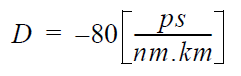Dispersion slope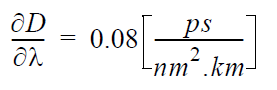Nonlinear coefficient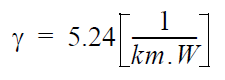Linear losses

α = 0.5 dB/km

Fiber length

LDCF = 10 km

After each segment from DCF, an amplifier compensates the linear losses.

Properties of Bessel optical filter are carrier wavelength &Lambda =1550 nm and bandwidth = 4 x Bit rate.

The cutoff frequency of the low pass Bessel electrical filter is 0.75 x Bit rate.

Ideal periodical amplification is performed with the help of the OptiSystem Ideal EDFA component, which also takes ASE noise into account. The amplifier after SMF has a gain of 10 dB and noise figure of 6 dB. (This after DCF with 5 dB gain and the same noise figure.)

Note the complete dispersion compensation of the GVD of the standard-mode fiber and the complete compensation of the power losses in both fibers. The post- compensation scheme for the dispersion compensation is used. Some of above parameters are chosen in accordance with , which allows comparison of results.

In the first part of this lesson, the comparison of RZ and NRZ formats was observed. Figure 3 shows the results obtained with high-dispersion fibers 40 Gbps in SMF RZ.osd for 40 Gb/s RZ modulation format (duty ratio = 0.5) transmission over common distance: from 500 km SMF (10 loops x 50 km) and periodical amplification after each fiber. The dependence of the max Q from the input power is shown in the graph. The BER Analyzer eye diagram represents the optimal point, which according to the graph, is input power ~ 4 mW.

Figure 3: 40 Gbps in SMF RZ results

A well-expressed maximum in the first graph can be seen.

Note: We have not performed any fitting of this curve.

At distances greater than 500 km, max Q becomes smaller than 6. Therefore, this is the maximum distance with good Q performance.

Figure 4 shows the results obtained with high-dispersion fibers 40 Gbps in SMF NRZ.osd for 40 Gb/s NRZ modulation format transmission over common distance from 250 km SMF (5 loops x 50 km) and periodical amplification after each fiber. The dependence of the max Q from the input power is shown in the graph. The BER Analyzer eye diagram represents the optimal point, which according to the graph, is input power ~ 1.25 mW.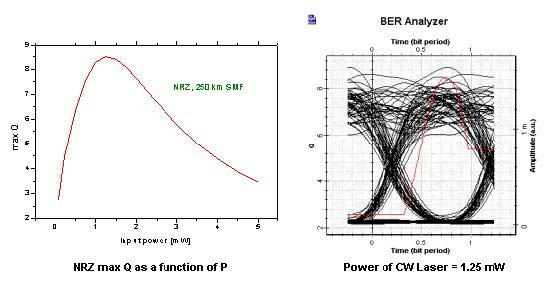Figure 4: 40 Gbps in SMF NRZ results

A well-expressed maximum in the first graph can be seen.

Note: We have not performed any fitting of this curve.

At distances greater than 250 km, max Q becomes smaller than 6. Therefore, this is the maximum available distance with good Q performance.

Comparing positions of the maximums of the two curves, we can clearly see the shift toward greater input powers for the RZ format. The results show that the RZ- modulation format duty cycle = 0.5 is superior to the conventional NRZ- modulation format.

In the second part of this lesson, the influence of accumulated amplifier noise and self-phase modulation on the RZ-modulation transmission in SMF at 40 Gb/s will be analyzed. Two different situations will be considered:

• self-phase modulation is assumed zero
• noise figure is not taken into account

Figure 5 shows the results.Figure 5: Transmission distance 500 km at 40 Gb/s

At low power levels, the performance is mainly hampered by the accumulated amplifier noise. At high input power levels, the transmission distance is significantly reduced due to the self-phase modulation. Just these effects determine the well- expressed maximum in the Q curve in the first figure.

In the third part of this lesson, two dispersion compensation schemes will be studied

(with high-dispersion fibers 40 Gbps in SMF RZ pre):

• post-compensation
• pre -compensation

Figure 6 shows the project layout.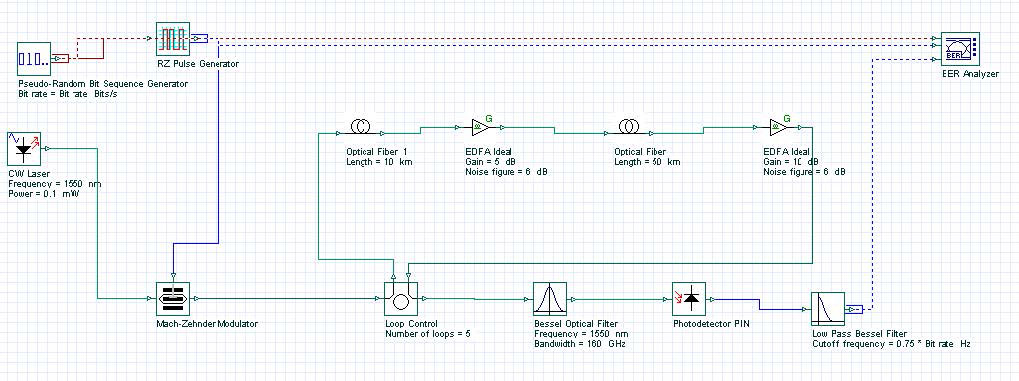Figure 6: 40 Gbps in SMF RZ pre-compensation layout

Figure 7 shows the corresponding results for the RZ-modulation transmission in SMF at 40 Gb/s.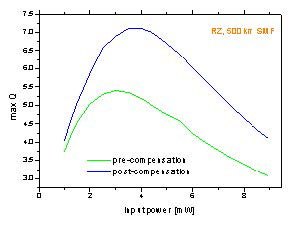Figure 7: RZ modulation format transmission at 40 Gb/s over 500 km in SMF

The results indicate that the post-compensation scheme is superior compared to the pre-compensation scheme in dispersion compensated systems at 40 Gb/s in SMF.

This lesson demonstrated that for upgrading the existing standard fiber network at

1.55 mm at 40 Gbps:

• Dispersion compensation is necessary
• RZ-modulation is superior compared to conventional NRZ-modulation format
• At low power levels, the performance is mainly hampered by the accumulated amplification
• At higher input powers, the transmission distance is significantly reduced by nonlinear self-phase modulation
• Post-compensation scheme is superior compared to pre-compensation scheme. The results agree well with the results of , , and .

References:

1      D. Breuer and K. Petermann, “Comparison of NRZ and RZ- Modulation Format for 40 Gbit/s TDM Standard-Fiber System,” IEEE Photonics Technology Letters, Vol. 9, pp. 398-400, 1997.

2      M.I. Hayee and A.E. Willner, “NRZ Versus RZ in 10-40 Gb/s Dispersion – Managed WDM Transmission Systems,” IEEE Photonics Technology Letters, Vol. 11, pp. 991-993, 1999.

3      C.M. Weinert, R. Ludvig, W. Papier, H.G. Weber, D. Breuer, K. Petermann, and F. Kuppers, “40 Gbit/s Comparison and 4 x 40 Gbit/s TDM/WDM Standard Fiber Transmission”, Journal of Lightwave Technology, Vol. 17, pp. 2276-2284, 1999.